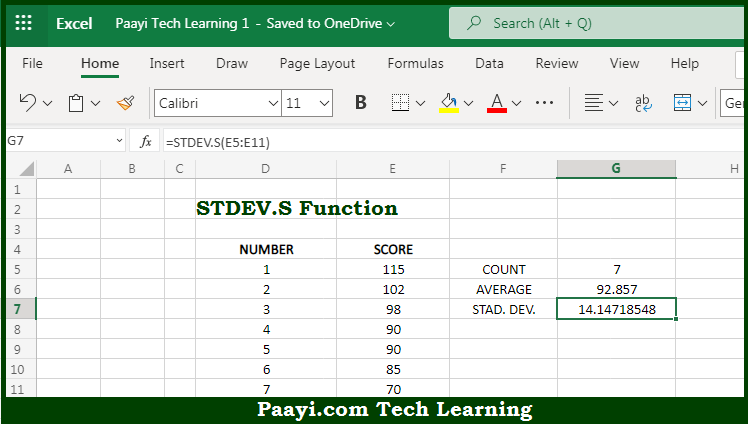# Learn How to Use Microsoft Excel STDEV.S Function

Written by | 0 Comments | 566 Views

In this article, you will learn how to use the Microsoft Excel STDEV.S function and its prime function in Microsoft Excel. You will also get to know the Microsoft Excel STDEV.S function return value and syntax with the help of some examples.

Microsoft Excel STDEV.S Function

The main purpose of the Microsoft Excel STDEV.S function is to get the standard deviation in the population. That implies, with the help of the STDEV.S function you can able to return the standard deviation for data that represents a sample or population. It should be noted that the STDEV.S function replaces the older STDEV function, with the same behavior. So, with the help of the STDEV.S function, you can able to get the standard deviation in the given sample or population.

Return Value of STDEV.S Function

The return value will be the estimated standard deviation.

Syntax of STDEV.S Function

=STDEV.S(number1, [number2], ...)

Where the arguments:

• number1: This is the first number or reference in the sample.
• number2: This is the second number or reference in the sample (optional).

How to Use Microsoft Excel STDEV.S Function?So we know that Microsoft Excel STDEV.S function you can able to get the standard deviation in the population. That implies, with the help of the STDEV.S function you can able to return the standard deviation for data that represents a sample or population. It should be noted that the STDEV.S function replaces the older STDEV function, with the same behavior. So, with the help of the STDEV.S function, you can able to get the standard deviation in the given sample or population.